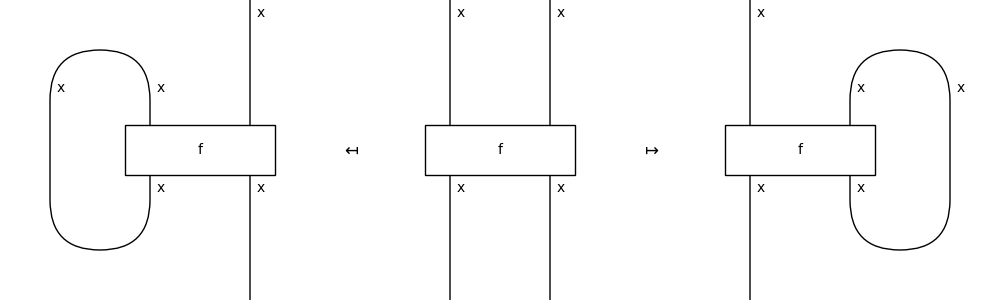# Diagram#

class discopy.traced.Diagram(inside, dom, cod, _scan=True)[source]#

Bases: `Diagram`

A traced diagram is a monoidal diagram with `Trace` boxes.

Parameters:
• inside (monoidal.Layer) – The layers inside the diagram.

• dom (monoidal.Ty) – The domain of the diagram, i.e. its input.

• cod (monoidal.Ty) – The codomain of the diagram, i.e. its output.

trace(n=1, left=False)[source]#

Feed `n` outputs back into inputs.

Parameters:
• n – The number of output wires to feedback into inputs.

• left – Whether to trace the wires on the left or right.

Example

```>>> from discopy.drawing import Equation as Eq
>>> x = Ty('x')
>>> f = Box('f', x @ x, x @ x)
>>> LHS, RHS = f.trace(left=True), f.trace(left=False)
>>> Eq(Eq(LHS, f, symbol="\$\\mapsfrom\$"),
...     RHS, symbol="\$\\mapsto\$").draw(
...         path="docs/_static/traced/trace.png")
```factory#

alias of `Diagram`

trace_factory#

alias of `Trace`